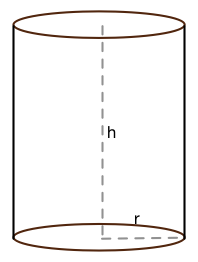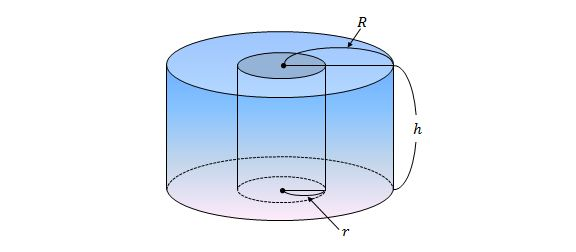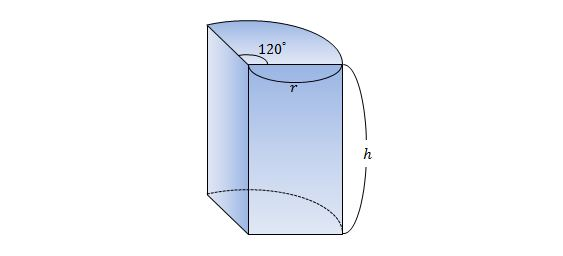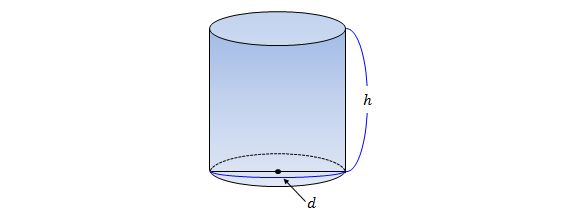Geometry

Volume - CylinderIf a cylinder has radius 3, and height $30$, what is its volume?

If we have a cylinder with a circumference of $6$ and a height of $3$, what is the volume of the cylinder?The above diagram shows a hollow cylinder. If $r=2,$ $R=5$ and $h=7,$ what is the volume of the body?If $r=6$ and $h=6,$ what is the volume of the above body?If $d=10$ and $h=14$ in the above diagram, what is the volume of the cylinder?

×